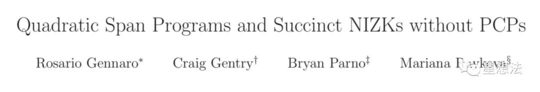## 背景介绍

QSP/QAP 问题的思想都是出自 2012 年一篇论文：Quadratic Span Programs and Succinct NIZKs without PCPs## 术语介绍

• SP - Span Program ，采用多项式形式实现计算的验证。
• QSP - Quadratic Span Program，QSP 问题，实现基于布尔电路的 NP 问题的证明和验证。
• QAP - Quadratic Arithmetic Program，QAP 问题，实现基于算术电路的 NP 问题的证明和验证，相对于 QSP，QAP 有更好的普适性。
• PCP - Probabilistically Checkable Proof ，在 QSP 和 QAP 理论之前，学术界主要通过 PCP 理论实现计算验证。PCP 是一种基于交互的，随机抽查的计算验证系统。
• NIZK - Non-Interactive Zero-Knowledge，统称，无交互零知识验证系统。NIZK 需要满足三个条件：1/ 完备性(Completeness)，对于正确的解，肯定存在相应证明。 2/可靠性 (Soundness) ，对于错误的解，能通过验证的概率极低。3/ 零知识。
• SNARG - Succinct Non-interactive ARGuments，简洁的无须交互的证明过程。
• SNARK - Succinct Non-interactive ARgumentss of Knowledge，相比 SNARG，SNARK 多了 Knowledge，也就是说，SNARK 不光能证明计算过程，还能确认证明者“拥有”计算需要的 Knowledge（只要证明者能给出证明就证明证明者拥有相应的解）。
• zkSNARK - zero-knowledge SNARK，在 SNARK 的基础上，证明和验证双方除了能验证计算外，验证者对其他信息一无所知。
• Statement - 对于 QSP/QAP，和电路结构本身（计算函数）相关的参数。比如说，某个计算电路的输入/输出以及电路内部门信息。Statement 对证明者和验证者都是公开的。
• Witness - Witness 只有证明者知道。可以理解成，某个计算电路的正确的解（输入）。

## QAP 问题的定义

QAP 的定义和 QSP 的定义有些相似（毕竟都是一个思想理论的两种形式）。论文中给出了 QAP 的一般定义和强定义。QAP 的强定义如下：

QAP 问题是这样一个 NP 问题：给定一系列的多项式，以及给定一个目标多项式，找出多项式的组合能整除目标多项式。输入为 n 位的 QAP 问题定义如下：

• 给定多个多项式： $v_0, ... , v_m, w_0, ... , w_m, y_0, ... , y_m$
• 目标多项式： $t$
• 映射函数： $$f: \left{(i, j) |1\leq i \leq n, j\in{0,1} \right} \to \left{1, ... m\right}$$

• $$a_k, b_k, c_k = 1\ \ 如果 k = f(i, u[i])$$
• $$a_k, b_k, c_k = 0\ \ 如果 k = f(i, 1- u[i])$$
• $$(v0(x) + \sum{k=1}^m a_k \cdot v_k(x)) \cdot (w0(x) + \sum{k=1}^m b_k \cdot w_k(x)) - (y0(x) + \sum{k=1}^m c_k \cdot y_k(x)) 能整除 t(x)$$

## QAP 问题的转化

### 门描述

$$s \cdot a* s \cdot b - s \cdot c = 0$$

$a = [0, 1, 0, 0, 0, 0]$

$b = [0, 1, 0, 0, 0, 0]$

$c = [0, 0, 0, 1, 0, 0]$

$a = [0, 0, 0, 1, 0, 0]$

$b = [0, 1, 0, 0, 0, 0]$

$c = [0, 0, 0, 0, 1, 0]$

$a = [0, 1, 0, 0, 1, 0]$

$b = [1, 0, 0, 0, 0, 0]$

$c = [0, 0, 0, 0, 0, 1]$

$a = [5, 0, 0, 0, 0, 1]$

$b = [1, 0, 0, 0, 0, 0]$

$c = [0, 0, 1, 0, 0, 0]$

### 多项式表达

$f_0(1) = 0, f_0(2) = 0, f_0(3)=0, f_0(4)=5$

$$s \cdot a* s \cdot b - s \cdot c = 0$$

，在正确的 $s$ 向量值的情况下，1/2/3/4 能让等式成立，也就是说，多项式 $s \cdot a* s \cdot b - s \cdot c$ 能整除 $(x-1)(x-2)(x-3)(x-4)$ 。这样，一个算术电路就转化为了 QAP 问题。

## QAP 问题的 zkSNARK 证明

QAP 问题的 zkSNARK 证明过程和 QSP 有点类似。skSNARK 证明过程分为两部分：a) setup 阶段 b）证明阶段。QAP 问题就是给定一系列的多项式 $v_0, ..., v_m, w_0, ..., w_m, y_0, ... , y_m$ 以及目标多项式 $t$ ，证明存在一个证据 $u$ 。这些多项式中的最高阶为 $d$ 。

### setup 和 CRS

CRS - Common Reference String，也就是预先 setup 的公开信息。在选定 $s$ 和 $\alpha$ 的情况下，发布如下信息：

• $s$ 和 $\alpha$ 的计算结果

$(s^0), E(s^1), ... , E(s^d)$

$E(\alpha s^0), E(\alpha s^1), ... , E(\alpha s^d)$

• 多项式的 $\alpha$ 对的计算结果 $E(t(s)), E(\alpha t(s))$

$E(v_0(s)), ... E(v_m(s)), E(\alpha v_0(s)), ..., E(\alpha v_m(s))$

$E(w_0(s)), ... E(w_m(s)), E(\alpha w_0(s)), ..., E(\alpha w_m(s))$

$E(y_0(s)), ... E(y_m(s)), E(\alpha y_0(s)), ..., E(\alpha y_m(s))$

• 多项式的 $\beta_v, \beta_w, \beta_y, \gamma$ 参数的计算结果 $E(\gamma), E(\beta_v\gamma), E(\beta_w\gamma), E(\beta_y\gamma)$

$E(\beta_vv_1(s)), ... , E(\beta_vv_m(s))$

$E(\beta_ww_1(s)), ... , E(\beta_ww_m(s))$

$E(\beta_yy_1(s)), ... , E(\beta_yy_m(s))$

$E(\beta_vt(s)), E(\beta_wt(s)), E(\beta_yt(s))$

### 证明者提供证据

$v_{free}(x) = \sum_k a_kv_k(x)$

• $V{free} := E(v{free}(s)), \ W := E(w(s)), \ Y := E(y(s)), \ H := E(h(s)),$
• $V{free}' := E(\alpha v{free}(s)), W' := E(\alpha w(s)), Y' := E(\alpha y(s)), H' := E(\alpha h(s)),$
• $P := E(\betavv{free}(s) + \beta_ww(s) + \beta_yy(s))$

$V{free}/V{free}', W/W', Y/Y', H/H' 是 \alpha 对，用以验证v{free},w,y,h 是否是多项式形式。$ $t 是已知，公开的，毋需验证， P 用来确保 v{free}(s), w(s) 和 y(s)的计算采用一致的参数。$

### 验证者验证

$v_{in}(x) = \sum_k a_kv_k(x)$

• $e(V{free}', g) = e(V{free}, g^\alpha), e(W', E(1)) = e(W, E(\alpha)), e(Y', E(1)) = e(Y, E(\alpha)), e(H', E(1)) = e(H, E(\alpha))$
• $e(E(\gamma), P) = e(E(\betav\gamma), V{free})e(E(\beta_w\gamma), W)e(E(\beta_y\gamma), Y)$
• $e(E(v0(s))E(v{in}(s))V_{free}, E(w_0(s))W) = e(H, E(t(s)))e(y_0(s)Y, E(1))$

$e(E(\gamma), P) = e(E(\gamma), E(\betavv{free}(s) + \beta_ww(s) + \beta_yy(s))) = e(g, g)^{\gamma(\betavv{free}(s) + \beta_ww(s) + \beta_yy(s))}$

$e(E(\betav\gamma), V{free})e(E(\beta_w\gamma), W)e(E(\beta_y\gamma), Y) = e(E(\betav\gamma), E(v{free}(s)))e(E(\beta_w\gamma), E(w(s)))e(E(\beta_y\gamma), E(y(s)))$

$= e(g,g)^{(\betav\gamma)v{free}(s)}e(g,g)^{(\beta_w\gamma)w(s)}e(g,g)^{(\beta_y\gamma)y(s)}= e(g, g)^{\gamma(\betavv{free}(s) + \beta_ww(s) + \beta_yy(s))}$

### $\delta$ 偏移

$v{free}(s) \rightarrow v{free}(s) + \delta_{free}t(s)$ $w(s) \rightarrow w(s) + \delta_wt(s)$ $y(s) \rightarrow y(s) + \deltayt(s)$ $h(s) \rightarrow h(s)+\delta{free}(w_0(s) + w(s)) + \delta_w(v0(s) + v{in}(s) + v{free}(s)) + (\delta{free}\delta_w)t(s) - \delta_y$

## 总结：

QAP和QSP问题类似。QSP问题主要用于布尔电路计算表达，QAP问题主要用于算术电路计算表达。将一个算术电路计算转化为QAP问题的过程，其实就是对电路中每个门电路进行描述限制的过程。通过朗格朗日定理，实现算术电路的多项式表达。QAP问题的zkSNARK的证明验证过程和QSP非常相似。• 发表于 2019-05-07 15:10
• 阅读 ( 7778 )
• 学分 ( 20 )
• 分类：隐私